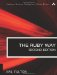# Section 5.26. Variance and Standard Deviation

### 5.25. Finding the Mean, Median, and Mode of a Data Set

Given an array x, let's find the mean of all the values in that array. Actually, there are three common kinds of mean. The ordinary or arithmetic mean is what we call the average in everyday life. The harmonic mean is the number of terms divided by the sum of all their reciprocals. And finally, the geometric mean is the nth root of the product of the n values. We show each of these in the following example:

`def mean(x)   sum=0   x.each {|v| sum += v}   sum/x.size end def hmean(x)   sum=0   x.each {|v| sum += (1.0/v)}   x.size/sum end def gmean(x)   prod=1.0   x.each {|v| prod *= v}   prod**(1.0/x.size) end data = [1.1, 2.3, 3.3, 1.2, 4.5, 2.1, 6.6] am = mean(data)   # 3.014285714 hm = hmean(data)  # 2.101997946 gm = gmean(data)  # 2.508411474`

The median value of a data set is the value that occurs approximately in the middle of the (sorted) set. (The following code fragment computes a median.) For this value, half the numbers in the set should be less, and half should be greater. Obviously, this statistic will be more appropriate and meaningful for some data sets than others. See the following code:

`def median(x)   sorted = x.sort   mid = x.size/2   sorted[mid] end data = [7,7,7,4,4,5,4,5,7,2,2,3,3,7,3,4] puts median(data)          # 4`

The mode of a data set is the value that occurs most frequently. If there is only one such value, the set is unimodal; otherwise, it is multimodal. A multimodal data set is a more complex case that we do not consider here. The interested reader can extend and improve the code we show here:

`def mode(x)    f = {}      # frequency table    fmax = 0    # maximum frequency    m = nil     # mode    x.each do |v|      f[v] ||= 0      f[v] += 1      fmax,m = f[v], v if f[v] > fmax    end    return m end data = [7,7,7,4,4,5,4,5,7,2,2,3,3,7,3,4] puts mode(data)            # 7`The Ruby Way, Second Edition: Solutions and Techniques in Ruby Programming (2nd Edition)
ISBN: 0672328844
EAN: 2147483647
Year: 2004
Pages: 269
Authors: Hal Fulton

Similar book on Amazon

flylib.com © 2008-2017.
If you may any questions please contact us: flylib@qtcs.net